# Observable Factory¶

reactivex.amb(*sources)

Propagates the observable sequence that emits first.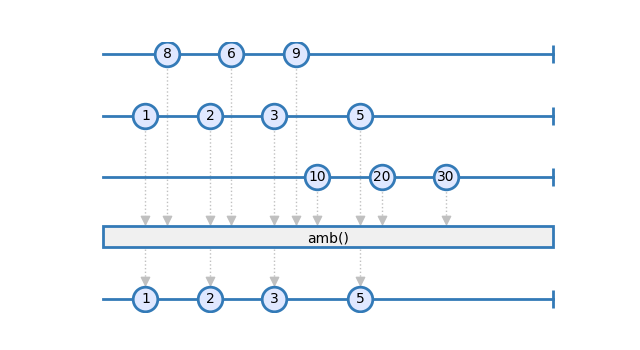Example

```>>> winner = reactivex.amb(xs, ys, zs)
```
Parameters

sources (`Observable`[`TypeVar`(`_T`)]) – Sequence of observables to monitor for first emission.

Return type

`Observable`[`TypeVar`(`_T`)]

Returns

An observable sequence that surfaces any of the given sequences, whichever emitted the first element.

reactivex.case(mapper, sources, default_source=None)

Uses mapper to determine which source in sources to use.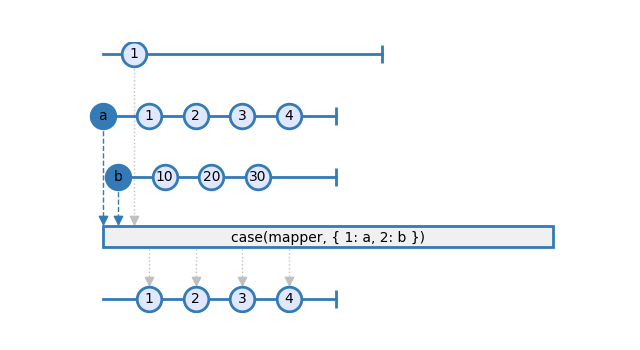Examples

```>>> res = reactivex.case(mapper, { '1': obs1, '2': obs2 })
>>> res = reactivex.case(mapper, { '1': obs1, '2': obs2 }, obs0)
```
Parameters
• mapper – The function which extracts the value for to test in a case statement.

• sources – An object which has keys which correspond to the case statement labels.

• default_source – [Optional] The observable sequence or Future that will be run if the sources are not matched. If this is not provided, it defaults to `empty()`.

Returns

An observable sequence which is determined by a case statement.

reactivex.catch(*sources)

Continues observable sequences which are terminated with an exception by switching over to the next observable sequence.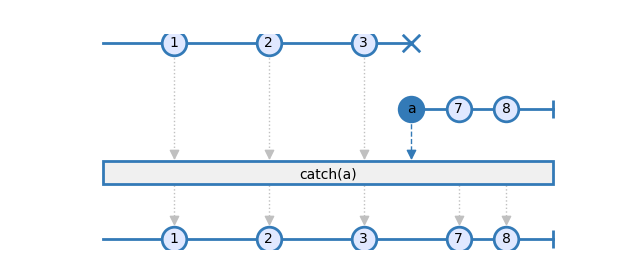Examples

```>>> res = reactivex.catch(xs, ys, zs)
```
Parameters

sources (`Observable`[`TypeVar`(`_T`)]) – Sequence of observables.

Return type

`Observable`[`TypeVar`(`_T`)]

Returns

An observable sequence containing elements from consecutive observables from the sequence of sources until one of them terminates successfully.

reactivex.catch_with_iterable(sources)

Continues observable sequences that are terminated with an exception by switching over to the next observable sequence.Examples

```>>> res = reactivex.catch([xs, ys, zs])
>>> res = reactivex.catch(src for src in [xs, ys, zs])
```
Parameters

sources (`Iterable`[`Observable`[`TypeVar`(`_T`)]]) – An Iterable of observables; thus, a generator can also be used here.

Return type

`Observable`[`TypeVar`(`_T`)]

Returns

An observable sequence containing elements from consecutive observables from the sequence of sources until one of them terminates successfully.

reactivex.create(subscribe)
Creates an observable sequence object from the specified

subscription function.Parameters

subscribe (`Callable`[[`ObserverBase`[`TypeVar`(`_T`)], `Optional`[`SchedulerBase`]], `DisposableBase`]) – Subscription function.

Return type

`Observable`[`TypeVar`(`_T`)]

Returns

An observable sequence that can be subscribed to via the given subscription function.

reactivex.combine_latest(__a: Observable[_A], __b: Observable[_B]) Observable[Tuple[_A, _B]]
reactivex.combine_latest(__a: Observable[_A], __b: Observable[_B], __c: Observable[_C]) Observable[Tuple[_A, _B, _C]]
reactivex.combine_latest(__a: Observable[_A], __b: Observable[_B], __c: Observable[_C], __d: Observable[_D]) Observable[Tuple[_A, _B, _C, _D]]

Merges the specified observable sequences into one observable sequence by creating a tuple whenever any of the observable sequences emits an element.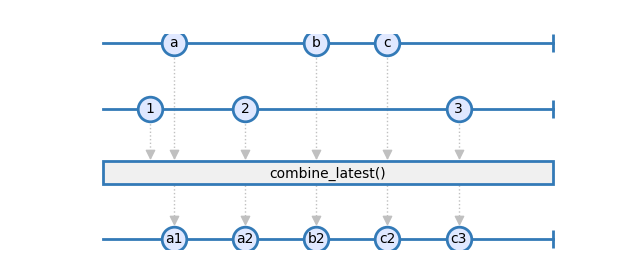Examples

```>>> obs = rx.combine_latest(obs1, obs2, obs3)
```
Parameters

sources – Sequence of observables.

Return type

`Observable`[`Any`]

Returns

An observable sequence containing the result of combining elements from each source in given sequence.

reactivex.compose(*operators)

Compose multiple operators left to right.

Composes zero or more operators into a functional composition. The operators are composed to left to right. A composition of zero operators gives back the source.

Examples

```>>> pipe()(source) == source
>>> pipe(f)(source) == f(source)
>>> pipe(f, g)(source) == g(f(source))
>>> pipe(f, g, h)(source) == h(g(f(source)))
...
```
Return type

`Callable`[[`Any`], `Any`]

Returns

The composed observable.

reactivex.concat(*sources)

Concatenates all of the specified observable sequences.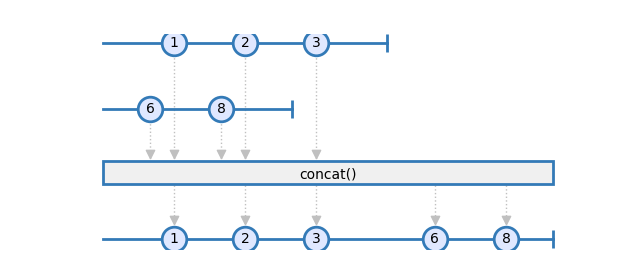Examples

```>>> res = reactivex.concat(xs, ys, zs)
```
Parameters

sources (`Observable`[`TypeVar`(`_T`)]) – Sequence of observables.

Return type

`Observable`[`TypeVar`(`_T`)]

Returns

An observable sequence that contains the elements of each source in the given sequence, in sequential order.

reactivex.concat_with_iterable(sources)

Concatenates all of the specified observable sequences.Examples

```>>> res = reactivex.concat_with_iterable([xs, ys, zs])
>>> res = reactivex.concat_with_iterable(for src in [xs, ys, zs])
```
Parameters

sources (`Iterable`[`Observable`[`TypeVar`(`_T`)]]) – An Iterable of observables; thus, a generator can also be used here.

Return type

`Observable`[`TypeVar`(`_T`)]

Returns

An observable sequence that contains the elements of each given sequence, in sequential order.

class reactivex.ConnectableObservable(source, subject)

Represents an observable that can be connected and disconnected.

__init__(source, subject)

Creates an observable sequence object from the specified subscription function.

Parameters

subscribe – [Optional] Subscription function

connect(scheduler=None)

Connects the observable.

Return type

`Optional`[`DisposableBase`]

auto_connect(subscriber_count=1)

Returns an observable sequence that stays connected to the source indefinitely to the observable sequence. Providing a subscriber_count will cause it to connect() after that many subscriptions occur. A subscriber_count of 0 will result in emissions firing immediately without waiting for subscribers.

Return type

`Observable`[`TypeVar`(`_T`)]

reactivex.defer(factory)

Returns an observable sequence that invokes the specified factory function whenever a new observer subscribes.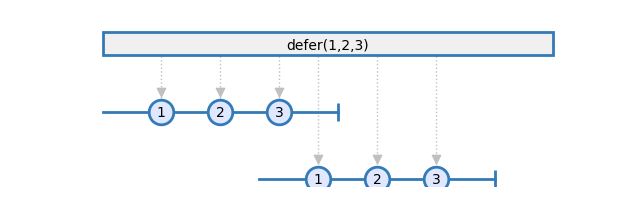Example

```>>> res = reactivex.defer(lambda scheduler: of(1, 2, 3))
```
Parameters

factory – Observable factory function to invoke for each observer which invokes `subscribe()` on the resulting sequence. The factory takes a single argument, the scheduler used.

Returns

An observable sequence whose observers trigger an invocation of the given factory function.

reactivex.empty(scheduler=None)

Returns an empty observable sequence.Example

```>>> obs = reactivex.empty()
```
Parameters

scheduler (`Optional`[`SchedulerBase`]) – [Optional] Scheduler instance to send the termination call on. By default, this will use an instance of `ImmediateScheduler`.

Return type

`Observable`[`Any`]

Returns

An observable sequence with no elements.

reactivex.fork_join(__a: Observable[_A], __b: Observable[_B]) Observable[Tuple[_A, _B]]
reactivex.fork_join(__a: Observable[_A], __b: Observable[_B], __c: Observable[_C]) Observable[Tuple[_A, _B, _C]]
reactivex.fork_join(__a: Observable[_A], __b: Observable[_B], __c: Observable[_C], __d: Observable[_D]) Observable[Tuple[_A, _B, _C, _D]]
reactivex.fork_join(__a: Observable[_A], __b: Observable[_B], __c: Observable[_C], __d: Observable[_D], __e: Observable[_E]) Observable[Tuple[_A, _B, _C, _D, _E]]

Wait for observables to complete and then combine last values they emitted into a tuple. Whenever any of that observables completes without emitting any value, result sequence will complete at that moment as well.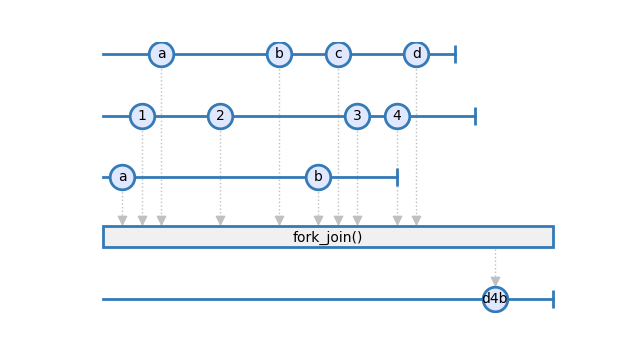Examples

```>>> obs = reactivex.fork_join(obs1, obs2, obs3)
```
Parameters

sources (`Observable`[`Any`]) – Sequence of observables.

Return type

`Observable`[`Any`]

Returns

An observable sequence containing the result of combining last element from each source in given sequence.

reactivex.from_callable(supplier, scheduler=None)

Returns an observable sequence that contains a single element generated by the given supplier, using the specified scheduler to send out observer messages.Examples

```>>> res = reactivex.from_callable(lambda: calculate_value())
>>> res = reactivex.from_callable(lambda: 1 / 0) # emits an error
```
Parameters
• supplier (`Callable`[[], `TypeVar`(`_T`)]) – Function which is invoked to obtain the single element.

• scheduler (`Optional`[`SchedulerBase`]) – [Optional] Scheduler instance to schedule the values on. If not specified, the default is to use an instance of `CurrentThreadScheduler`.

Return type

`Observable`[`TypeVar`(`_T`)]

Returns

An observable sequence containing the single element obtained by invoking the given supplier function.

reactivex.from_callback(func, mapper=None)

Converts a callback function to an observable sequence.

Parameters
• func (`Callable`[`...`, `Callable`[`...`, `None`]]) – Function with a callback as the last argument to convert to an Observable sequence.

• mapper (`Optional`[`Callable`[[`Any`], `Any`]]) – [Optional] A mapper which takes the arguments from the callback to produce a single item to yield on next.

Return type

`Callable`[[], `Observable`[`Any`]]

Returns

A function, when executed with the required arguments minus the callback, produces an Observable sequence with a single value of the arguments to the callback as a list.

reactivex.from_future(future)

Converts a Future to an Observable sequenceParameters

future – A Python 3 compatible future. https://docs.python.org/3/library/asyncio-task.html#future

Returns

An observable sequence which wraps the existing future success and failure.

reactivex.from_iterable(iterable, scheduler=None)

Converts an iterable to an observable sequence.Example

```>>> reactivex.from_iterable([1,2,3])
```
Parameters
• iterable (`Iterable`[`TypeVar`(`_T`)]) – An Iterable to change into an observable sequence.

• scheduler (`Optional`[`SchedulerBase`]) – [Optional] Scheduler instance to schedule the values on. If not specified, the default is to use an instance of `CurrentThreadScheduler`.

Return type

`Observable`[`TypeVar`(`_T`)]

Returns

The observable sequence whose elements are pulled from the given iterable sequence.

class reactivex.GroupedObservable(key, underlying_observable, merged_disposable=None)
__init__(key, underlying_observable, merged_disposable=None)

Creates an observable sequence object from the specified subscription function.

Parameters

subscribe – [Optional] Subscription function

reactivex.never()

Returns a non-terminating observable sequence, which can be used to denote an infinite duration (e.g. when using reactive joins).Return type

`Observable`[`Any`]

Returns

An observable sequence whose observers will never get called.

Represents a notification to an observer.

__init__()

Default constructor used by derived types.

accept(on_next, on_error=None, on_completed=None)

Invokes the delegate corresponding to the notification or an observer and returns the produced result.

Examples

```>>> notification.accept(observer)
```
Parameters
• on_next (`Union`[`Callable`[[`TypeVar`(`_T`)], `None`], `ObserverBase`[`TypeVar`(`_T`)]]) – Delegate to invoke for an OnNext notification.

• on_error (`Optional`[`Callable`[[`Exception`], `None`]]) – [Optional] Delegate to invoke for an OnError notification.

• on_completed (`Optional`[`Callable`[[], `None`]]) – [Optional] Delegate to invoke for an OnCompleted notification.

Return type

`None`

Returns

Result produced by the observation.

to_observable(scheduler=None)

Returns an observable sequence with a single notification, using the specified scheduler, else the immediate scheduler.

Parameters

scheduler (`Optional`[`SchedulerBase`]) – [Optional] Scheduler to send out the notification calls on.

Return type

`ObservableBase`[`TypeVar`(`_T`)]

Returns

An observable sequence that surfaces the behavior of the notification upon subscription.

equals(other)

Indicates whether this instance and a specified object are equal.

Return type

`bool`

__eq__(other)

Return self==value.

Return type

`bool`

__hash__ = None
reactivex.on_error_resume_next(*sources)

Continues an observable sequence that is terminated normally or by an exception with the next observable sequence.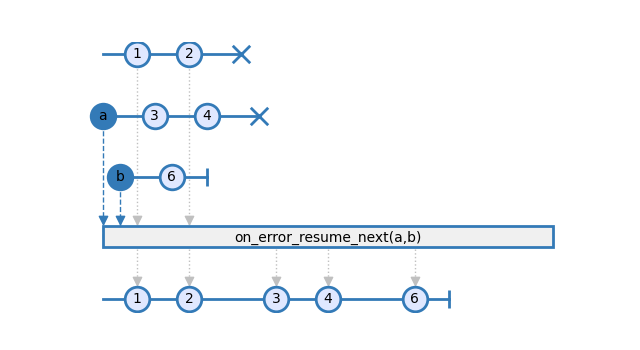Examples

```>>> res = reactivex.on_error_resume_next(xs, ys, zs)
```
Parameters

sources – Sequence of sources, each of which is expected to be an instance of either `Observable` or `Future`.

Returns

An observable sequence that concatenates the source sequences, even if a sequence terminates with an exception.

reactivex.of(*args)

This method creates a new observable sequence whose elements are taken from the arguments.Note

This is just a wrapper for `reactivex.from_iterable(args)`

Example

```>>> res = reactivex.of(1,2,3)
```
Parameters

args (`TypeVar`(`_T`)) – The variable number elements to emit from the observable.

Return type

`Observable`[`TypeVar`(`_T`)]

Returns

The observable sequence whose elements are pulled from the given arguments

class reactivex.Observable(subscribe=None)

Observable base class.

Represents a push-style collection, which you can `pipe` into `operators`.

__init__(subscribe=None)

Creates an observable sequence object from the specified subscription function.

Parameters

subscribe (`Optional`[`Callable`[[`ObserverBase`[`TypeVar`(`_T_out`, covariant=True)], `Optional`[`SchedulerBase`]], `DisposableBase`]]) – [Optional] Subscription function

subscribe(on_next=None, on_error=None, on_completed=None, *, scheduler=None)

Subscribe an observer to the observable sequence.

You may subscribe using an observer or callbacks, not both; if the first argument is an instance of `Observer` or if it has a (callable) attribute named `on_next`, then any callback arguments will be ignored.

Examples

```>>> source.subscribe()
>>> source.subscribe(observer)
>>> source.subscribe(observer, scheduler=scheduler)
>>> source.subscribe(on_next)
>>> source.subscribe(on_next, on_error)
>>> source.subscribe(on_next, on_error, on_completed)
>>> source.subscribe(on_next, on_error, on_completed, scheduler=scheduler)
```
Parameters

• on_error (`Optional`[`Callable`[[`Exception`], `None`]]) – [Optional] Action to invoke upon exceptional termination of the observable sequence.

• on_completed (`Optional`[`Callable`[[], `None`]]) – [Optional] Action to invoke upon graceful termination of the observable sequence.

• on_next (`Union`[`ObserverBase`[`TypeVar`(`_T_out`, covariant=True)], `Callable`[[`TypeVar`(`_T_out`, covariant=True)], `None`], `None`]) – [Optional] Action to invoke for each element in the observable sequence.

• scheduler (`Optional`[`SchedulerBase`]) – [Optional] The default scheduler to use for this subscription.

Return type

`DisposableBase`

Returns

Disposable object representing an observer’s subscription to the observable sequence.

pipe(__op1: Callable[[Observable[_T_out]], _A]) _A
pipe(__op1: Callable[[Observable[_T_out]], _A], __op2: Callable[[_A], _B]) _B
pipe(__op1: Callable[[Observable[_T_out]], _A], __op2: Callable[[_A], _B], __op3: Callable[[_B], _C]) _C
pipe(__op1: Callable[[Observable[_T_out]], _A], __op2: Callable[[_A], _B], __op3: Callable[[_B], _C], __op4: Callable[[_C], _D]) _D
pipe(__op1: Callable[[Observable[_T_out]], _A], __op2: Callable[[_A], _B], __op3: Callable[[_B], _C], __op4: Callable[[_C], _D], __op5: Callable[[_D], _E]) _E
pipe(__op1: Callable[[Observable[_T_out]], _A], __op2: Callable[[_A], _B], __op3: Callable[[_B], _C], __op4: Callable[[_C], _D], __op5: Callable[[_D], _E], __op6: Callable[[_E], _F]) _F
pipe(__op1: Callable[[Observable[_T_out]], _A], __op2: Callable[[_A], _B], __op3: Callable[[_B], _C], __op4: Callable[[_C], _D], __op5: Callable[[_D], _E], __op6: Callable[[_E], _F], __op7: Callable[[_F], _G]) _G

Compose multiple operators left to right.

Composes zero or more operators into a functional composition. The operators are composed from left to right. A composition of zero operators gives back the original source.

Examples

```>>> source.pipe() == source
>>> source.pipe(f) == f(source)
>>> source.pipe(g, f) == f(g(source))
>>> source.pipe(h, g, f) == f(g(h(source)))
```
Parameters

operators (`Callable`[[`Any`], `Any`]) – Sequence of operators.

Return type

`Any`

Returns

The composed observable.

run()

Run source synchronously.

Subscribes to the observable source. Then blocks and waits for the observable source to either complete or error. Returns the last value emitted, or throws exception if any error occurred.

Examples

```>>> result = run(source)
```
Raises
• SequenceContainsNoElementsError – if observable completes (on_completed) without any values being emitted.

• Exception – raises exception if any error (on_error) occurred.

Return type

`Any`

Returns

The last element emitted from the observable.

__await__()

Awaits the given observable.

Return type

`Generator`[`Any`, `None`, `TypeVar`(`_T_out`, covariant=True)]

Returns

The last item of the observable sequence.

Pythonic version of `concat`.

Example

```>>> zs = xs + ys
```
Parameters

other (`Observable`[`TypeVar`(`_T_out`, covariant=True)]) – The second observable sequence in the concatenation.

Return type

`Observable`[`TypeVar`(`_T_out`, covariant=True)]

Returns

Concatenated observable sequence.

Pythonic use of `concat`.

Example

```>>> xs += ys
```
Parameters

other (`Observable`[`TypeVar`(`_T_out`, covariant=True)]) – The second observable sequence in the concatenation.

Return type

`Observable`[`TypeVar`(`_T_out`, covariant=True)]

Returns

Concatenated observable sequence.

__getitem__(key)

Pythonic version of `slice`.

Slices the given observable using Python slice notation. The arguments to slice are start, stop and step given within brackets [] and separated by the colons :.

It is basically a wrapper around the operators `skip`, `skip_last`, `take`, `take_last` and `filter`.

The following diagram helps you remember how slices works with streams. Positive numbers are relative to the start of the events, while negative numbers are relative to the end (close) of the stream.

``` r---e---a---c---t---i---v---e---!
0   1   2   3   4   5   6   7   8
-8  -7  -6  -5  -4  -3  -2  -1   0
```

Examples

```>>> result = source[1:10]
>>> result = source[1:-2]
>>> result = source[1:-1:2]
```
Parameters

key (`Union`[`slice`, `int`]) – Slice object

Return type

`Observable`[`TypeVar`(`_T_out`, covariant=True)]

Returns

Sliced observable sequence.

Raises

TypeError – If key is not of type `int` or `slice`

class reactivex.Observer(on_next=None, on_error=None, on_completed=None)

Base class for implementations of the Observer class. This base class enforces the grammar of observers where OnError and OnCompleted are terminal messages.

__init__(on_next=None, on_error=None, on_completed=None)
on_next(value)

Notify the observer of a new element in the sequence.

Return type

`None`

on_error(error)

Notify the observer that an exception has occurred.

Parameters

error (`Exception`) – The error that occurred.

Return type

`None`

on_completed()

Notifies the observer of the end of the sequence.

Return type

`None`

dispose()

Disposes the observer, causing it to transition to the stopped state.

Return type

`None`

to_notifier()

Creates a notification callback from an observer.

Returns the action that forwards its input notification to the underlying observer.

Return type

`Callable`[[`Notification`[`TypeVar`(`_T_in`, contravariant=True)]], `None`]

as_observer()

Hides the identity of an observer.

Returns an observer that hides the identity of the specified observer.

Return type

`ObserverBase`[`TypeVar`(`_T_in`, contravariant=True)]

reactivex.return_value(value, scheduler=None)

Returns an observable sequence that contains a single element, using the specified scheduler to send out observer messages. There is an alias called ‘just’.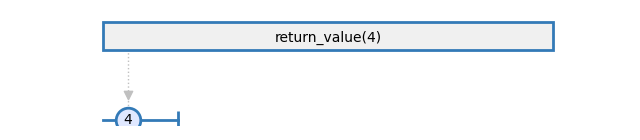Examples

```>>> res = reactivex.return_value(42)
>>> res = reactivex.return_value(42, timeout_scheduler)
```
Parameters

value (`TypeVar`(`_T`)) – Single element in the resulting observable sequence.

Return type

`Observable`[`TypeVar`(`_T`)]

Returns

An observable sequence containing the single specified element.

reactivex.pipe(__value, *fns)

Functional pipe (|>)

Allows the use of function argument on the left side of the function.

Example

```>>> pipe(x, fn) == __fn(x)  # Same as x |> fn
>>> pipe(x, fn, gn) == gn(fn(x))  # Same as x |> fn |> gn
...
```
Return type

`Any`

reactivex.range(start, stop=None, step=None, scheduler=None)

Generates an observable sequence of integral numbers within a specified range, using the specified scheduler to send out observer messages.Examples

```>>> res = reactivex.range(10)
>>> res = reactivex.range(0, 10)
>>> res = reactivex.range(0, 10, 1)
```
Parameters
• start (`int`) – The value of the first integer in the sequence.

• stop (`Optional`[`int`]) – [Optional] Generate number up to (exclusive) the stop value. Default is sys.maxsize.

• step (`Optional`[`int`]) – [Optional] The step to be used (default is 1).

• scheduler (`Optional`[`SchedulerBase`]) – [Optional] The scheduler to schedule the values on. If not specified, the default is to use an instance of `CurrentThreadScheduler`.

Return type

`Observable`[`int`]

Returns

An observable sequence that contains a range of sequential integral numbers.

reactivex.repeat_value(value, repeat_count=None)

Generates an observable sequence that repeats the given element the specified number of times.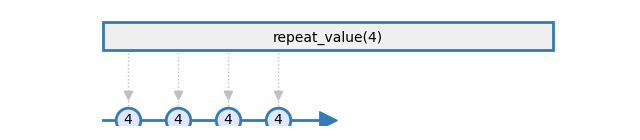Examples

```>>> res = reactivex.repeat_value(42)
>>> res = reactivex.repeat_value(42, 4)
```
Parameters
• value (`TypeVar`(`_T`)) – Element to repeat.

• repeat_count (`Optional`[`int`]) – [Optional] Number of times to repeat the element. If not specified, repeats indefinitely.

Return type

`Observable`[`TypeVar`(`_T`)]

Returns

An observable sequence that repeats the given element the specified number of times.

class reactivex.Subject

Represents an object that is both an observable sequence as well as an observer. Each notification is broadcasted to all subscribed observers.

__init__()

Creates an observable sequence object from the specified subscription function.

Parameters

subscribe – [Optional] Subscription function

on_next(value)

Notifies all subscribed observers with the value.

Parameters

value (`TypeVar`(`_T`)) – The value to send to all subscribed observers.

Return type

`None`

on_error(error)

Notifies all subscribed observers with the exception.

Parameters

error (`Exception`) – The exception to send to all subscribed observers.

Return type

`None`

on_completed()

Notifies all subscribed observers of the end of the sequence.

Return type

`None`

dispose()

Unsubscribe all observers and release resources.

Return type

`None`

reactivex.start(func, scheduler=None)

Invokes the specified function asynchronously on the specified scheduler, surfacing the result through an observable sequence.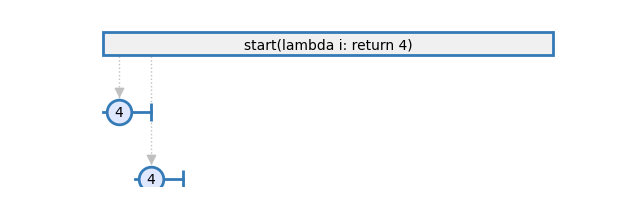Note

The function is called immediately, not during the subscription of the resulting sequence. Multiple subscriptions to the resulting sequence can observe the function’s result.

Example

```>>> res = reactivex.start(lambda: pprint('hello'))
>>> res = reactivex.start(lambda: pprint('hello'), rx.Scheduler.timeout)
```
Parameters
• func (`Callable`[[], `TypeVar`(`_T`)]) – Function to run asynchronously.

• scheduler (`Optional`[`SchedulerBase`]) – [Optional] Scheduler to run the function on. If not specified, defaults to an instance of `TimeoutScheduler`.

Return type

`Observable`[`TypeVar`(`_T`)]

Returns

An observable sequence exposing the function’s result value, or an exception.

reactivex.start_async(function_async)

Invokes the asynchronous function, surfacing the result through an observable sequence.Parameters

function_async – Asynchronous function which returns a `Future` to run.

Returns

An observable sequence exposing the function’s result value, or an exception.

reactivex.throw(exception, scheduler=None)

Returns an observable sequence that terminates with an exception, using the specified scheduler to send out the single OnError message.Example

```>>> res = reactivex.throw(Exception('Error'))
```
Parameters
• exception (`Union`[`str`, `Exception`]) – An object used for the sequence’s termination.

• scheduler (`Optional`[`SchedulerBase`]) – [Optional] Scheduler to schedule the error notification on. If not specified, the default is to use an instance of `ImmediateScheduler`.

Return type

`Observable`[`Any`]

Returns

The observable sequence that terminates exceptionally with the specified exception object.

reactivex.timer(duetime, period=None, scheduler=None)

Returns an observable sequence that produces a value after duetime has elapsed and then after each period.Examples

```>>> res = reactivex.timer(datetime(...))
>>> res = reactivex.timer(datetime(...), 0.1)
>>> res = reactivex.timer(5.0)
>>> res = reactivex.timer(5.0, 1.0)
```
Parameters
• duetime (`Union`[`datetime`, `timedelta`, `float`]) – Absolute (specified as a datetime object) or relative time (specified as a float denoting seconds or an instance of timedelta) at which to produce the first value.

• period (`Union`[`timedelta`, `float`, `None`]) – [Optional] Period to produce subsequent values (specified as a float denoting seconds or an instance of timedelta). If not specified, the resulting timer is not recurring.

• scheduler (`Optional`[`SchedulerBase`]) – [Optional] Scheduler to run the timer on. If not specified, the default is to use an instance of `TimeoutScheduler`.

Return type

`Observable`[`int`]

Returns

An observable sequence that produces a value after due time has elapsed and then each period.

reactivex.to_async(func, scheduler=None)

Converts the function into an asynchronous function. Each invocation of the resulting asynchronous function causes an invocation of the original synchronous function on the specified scheduler.Examples

```>>> res = reactivex.to_async(lambda x, y: x + y)(4, 3)
>>> res = reactivex.to_async(lambda x, y: x + y, Scheduler.timeout)(4, 3)
>>> res = reactivex.to_async(lambda x: log.debug(x), Scheduler.timeout)('hello')
```
Parameters
• func (`Callable`[`...`, `TypeVar`(`_T`)]) – Function to convert to an asynchronous function.

• scheduler (`Optional`[`SchedulerBase`]) – [Optional] Scheduler to run the function on. If not specified, defaults to an instance of `TimeoutScheduler`.

Return type

`Callable`[`...`, `Observable`[`TypeVar`(`_T`)]]

Returns

Asynchronous function.

reactivex.using(resource_factory, observable_factory)

Constructs an observable sequence that depends on a resource object, whose lifetime is tied to the resulting observable sequence’s lifetime.

Example

```>>> res = reactivex.using(lambda: AsyncSubject(), lambda: s: s)
```
Parameters
• resource_factory (`Callable`[[], `DisposableBase`]) – Factory function to obtain a resource object.

• observable_factory (`Callable`[[`DisposableBase`], `Observable`[`TypeVar`(`_T`)]]) – Factory function to obtain an observable sequence that depends on the obtained resource.

Return type

`Observable`[`TypeVar`(`_T`)]

Returns

An observable sequence whose lifetime controls the lifetime of the dependent resource object.

reactivex.with_latest_from(*sources)

Merges the specified observable sequences into one observable sequence by creating a `tuple` only when the first observable sequence produces an element.Examples

```>>> obs = rx.with_latest_from(obs1)
>>> obs = rx.with_latest_from([obs1, obs2, obs3])
```
Parameters

sources (`Observable`[`Any`]) – Sequence of observables.

Return type

`Observable`[`Tuple`[`Any`, `...`]]

Returns

An observable sequence containing the result of combining elements of the sources into a `tuple`.

reactivex.zip(*args)

Merges the specified observable sequences into one observable sequence by creating a `tuple` whenever all of the observable sequences have produced an element at a corresponding index.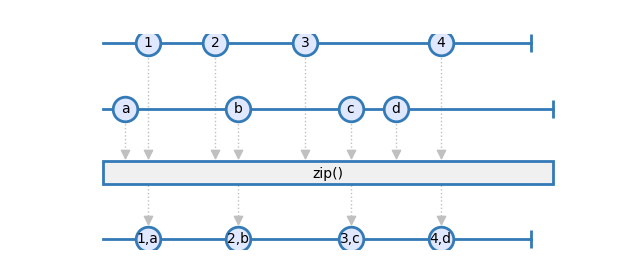Example

```>>> res = rx.zip(obs1, obs2)
```
Parameters

args (`Observable`[`Any`]) – Observable sources to zip.

Return type

`Observable`[`Tuple`[`Any`, `...`]]

Returns

An observable sequence containing the result of combining elements of the sources as a `tuple`.